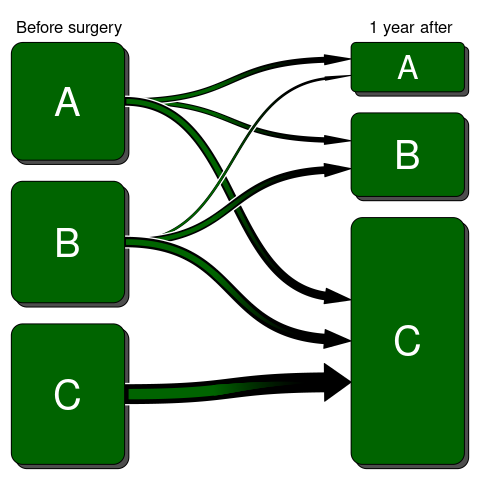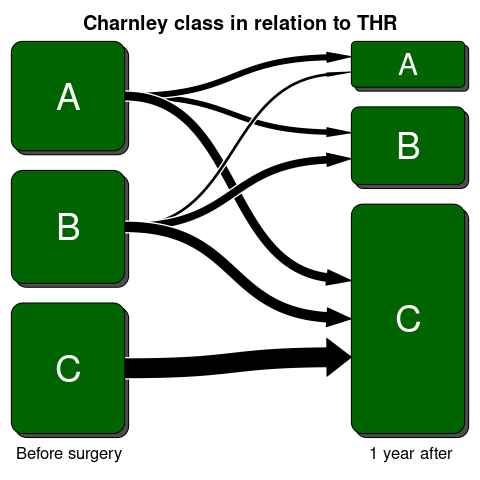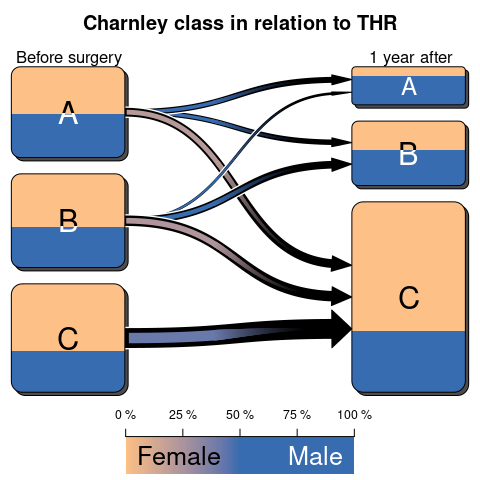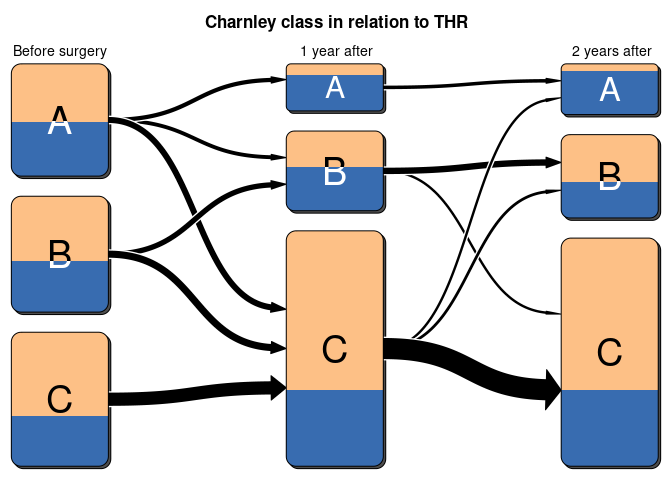# Introduction

The Transition-class is the advanced version of the transitionPlot that aims at illustrating transitions between classes over time. The original intent was to show how self-administered the Charnley classification behaves before and after surgery. The plot is a fancier version than what can be achieved using packages such as diagram, although it lacks some of the flexibility - most notable is the lack of ability to “go back in time”. Thus the current function only allows to show transitions from one state to the next and there is no going back to previous columns.

# Generate some data

We will start by simulating some data similar to my article. Each observation has a sex and a Charnley class (A, B, or C). The transition is then dependent on both the sex and the Charnley class.

set.seed(1)
library(magrittr)
n <- 100
data <-
data.frame(
Sex = sample(c("Male", "Female"),
size = n,
replace = TRUE,
prob = c(.4, .6)),
Charnley_class = sample(c("A", "B", "C"),
size = n,
replace = TRUE))
getProbs <- function(Chrnl_name){
prob <- data.frame(
A = 1/3 +
(data$Sex == "Male") * .25 + (data$Sex != "Male") * -.25 +
(data[[Chrnl_name]] %in% "B") * -.5 +
(data[[Chrnl_name]] %in% "C") * -2 ,
B = 1/3 +
(data$Sex == "Male") * .1 + (data$Sex != "Male") * -.05 +
(data[[Chrnl_name]] == "C") * -2,
C = 1/3 +
(data$Sex == "Male") * -.25 + (data$Sex != "Male") * .25)

# Remove negative probabilities
t(apply(prob, 1, function(x) {
if (any(x < 0)){
x <- x - min(x) + .05
}
x
}))
}

Ch_classes <- c("Charnley_class")
Ch_classes %<>% c(sprintf("%s_%dyr", Ch_classes, c(1,2,6)))
for (i in 1:length(Ch_classes)){
if (i == 1)
next;

data[[Ch_classes[i]]] <-
apply(getProbs(Ch_classes[i-1]), 1, function(p)
sample(c("A", "B", "C"),
size = 1,
prob = p)) %>%
factor(levels = c("A", "B", "C"))
}

# Basic use

The most simple use is to just supply the output from the table() call:

library(Gmisc)
transitions <- table(data$Charnley_class, data$Charnley_class_1yr) %>%
getRefClass("Transition")$new(label=c("Before surgery", "1 year after")) transitions$render()Adding customizations are rather straight forward by setting the different field arguments:

transitions <- table(data$Charnley_class, data$Charnley_class_1yr) %>%
getRefClass("Transition")$new(label=c("Before surgery", "1 year after")) transitions$title <- "Charnley class in relation to THR"
transitions$arrow_type <- "simple" transitions$box_label_pos <- "bottom"
transitions$render()## Adding a third dimension Underlying proportions can be visualized by splitting the box colors and blending the colors in the gradient arrows. The color blend is explained in the colorbar element at the bottom: transitions <- table(data$Charnley_class, data$Charnley_class_1yr, data$Sex) %>%
getRefClass("Transition")$new(label=c("Before surgery", "1 year after")) transitions$title <- "Charnley class in relation to THR"
transitions$clr_bar <- "bottom" transitions$render()# Multiple transitions

The major advantage with the Transition-class is that it allows for transitions at multiple time points. We do this by calling the addTransitions function as shown below:

transitions <- table(data$Charnley_class, data$Charnley_class_1yr, data$Sex) %>% getRefClass("Transition")$new(label=c("Before surgery", "1 year after"))
transitions$title <- "Charnley class in relation to THR" transitions$arrow_type <- "simple"
table(data$Charnley_class_1yr, data$Charnley_class_2yr, data$Sex) %>% transitions$addTransitions(label="2 years after")
library(grid)
transitions$max_lwd <- unit(.05, "npc") transitions$render()